# IFNA Function

Returns a specified alternate value if a formula results in an #N/A error

## What is the IFNA Function in Excel?

The IFNA function in Excel returns an alternate value as specified by the user if a formula results in an #N/A error. It is a Logical function that was introduced in MS Excel 2013. The purpose of the IFNA function is to use an alternate value instead of the #N/A error.

### Formula

=IFNA(value, value_if_na)

The IFNA function uses the following arguments:

1. Value (required argument) – This is the formula or expression to be checked for an #N/A error.
2. Value_if_na (required argument) – This provides the specified value if the result of the formula or expression is N/A error.

### How to use the IFNA Function in Excel?

It is a built-in function which can be used as a worksheet function in Excel. Let’s take an example:

Using the value in A3 and #N/A in A4, let’s see how to apply IFNA to get rid of #N/A.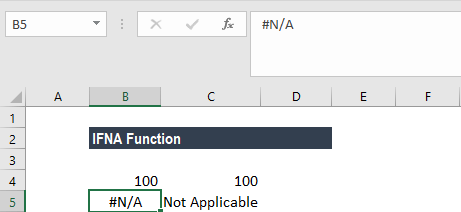When we use IFNA for both rows, the result would be: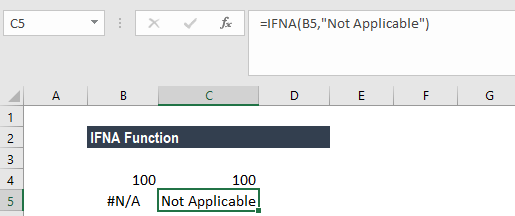### Uses of the IFNA Function in Excel

To understand the uses of the IFNA function, let’s consider a few examples:

#### Example 1

If we need to find out the prices of two commodities using the data below: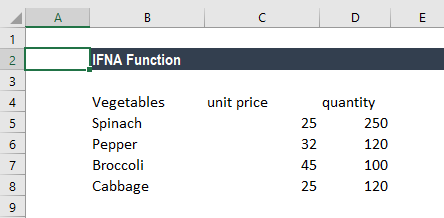Now we need to find out the price of Pepper and Banana from this information. If we just use the VLOOKUP formula, the result will be #N/A for Banana.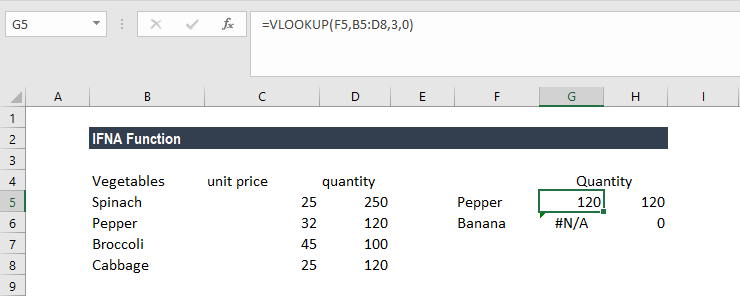However, if we use IFNA, we can instruct Excel to give us 0 instead of #N/A, as shown below: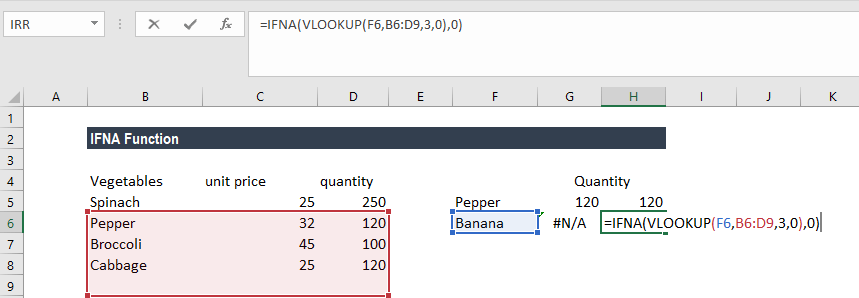The formula used here is: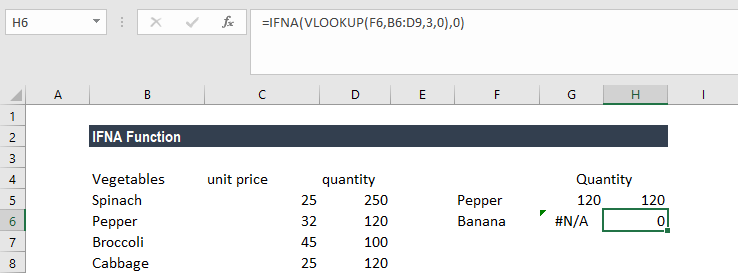Here, IFNA function tested the results of VLOOKUP function. VLOOKUP returned the #N/A error value as it couldn’t find Banana in the given lookup range. However, IFNA returned the value 0 in the cell instead of the standard #N/A error value.

On a similar basis, we can use the HLOOKUP formula combined with IFNA.

#### Example 2

As the IFNA function is more specific and targets only a specific error, which is #N/A, it is more appropriate to use than IFERROR function in certain circumstances. All other errors, such as #DIV/0! or #REF!, are ignored by the IFNA function. IFERROR, on the other hand, would trap other errors too.

Consider the data below: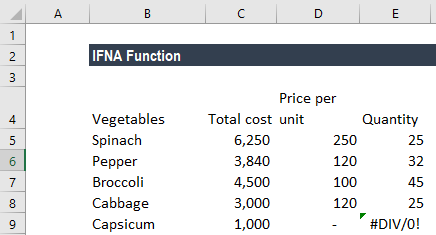The user made a typo error by putting 0 for the price per unit of capsicum. Now, when he wishes to derive the quantity for each vegetable, he would get a #DIV/0! error for the quantity of capsicum. Let’s see how the results would be different under IFNA function and IFERROR function.

When we use IFERROR formula as shown below, the result would be 0, as IFERROR considers all errors.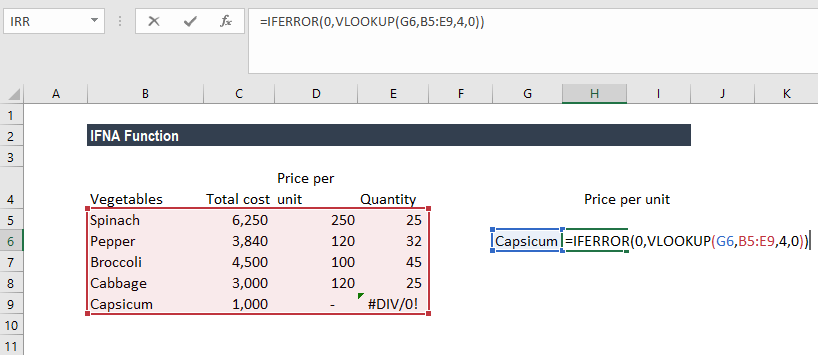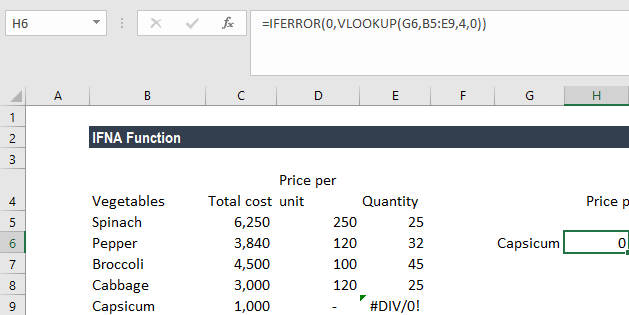Since the quantity is calculated by dividing the total price by price per unit, MS Excel would display the #DIV/0! error for vegetables whose prices were (erroneously) typed as 0 per unit.

This is a kind of error that we will handle in a different manner, compared to the way we will handle the #N/A error.

However, IFERROR considered it as an error and returned 0 for it.

On the other hand, IFNA would return #DIV/0! error as it considers only the #N/A error.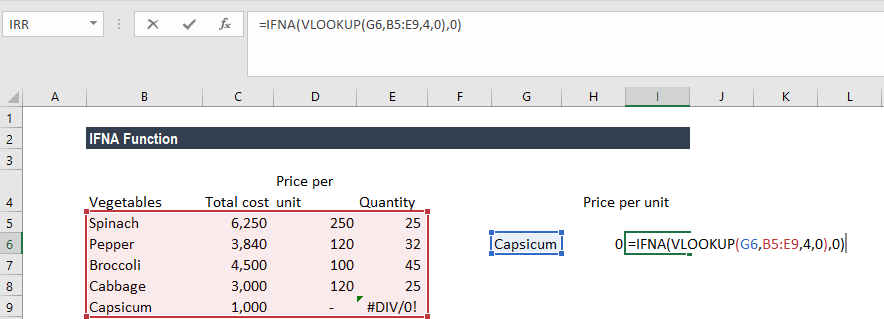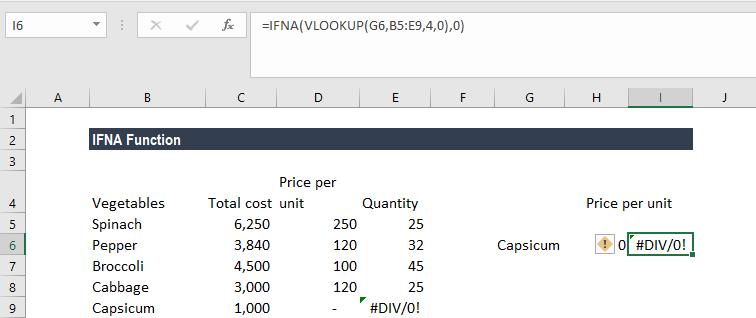So, IFNA becomes handy when you are only looking for the #N/A error only. The function becomes useful when we are handling extensive and complicated data sets where the user would miss different type of errors on the sheet.

### More on the IFNA Function in Excel

1. The IFNA function helps to trap error functions and is very useful when handling large amounts of data.
2. Remember that if the user provides value_if_na as an empty cell, the function would treat it as an empty string value (“”).
3. It is quicker when comes to troubleshooting, as it is more specific compared to IFERROR, ISERROR, ISERR, etc.

Thanks for reading CFI’s guide to important Excel functions! By taking the time to learn and master these functions, you’ll significantly speed up your financial analysis. To learn more, check out these additional CFI resources:

• Excel Functions for Finance